# 1. calendar处理日期

calendar模块第一了Calendar类，其中封装了一些值的计算，如给定的一个月或一年中的周日期。另外，TextCalendar和HTMLCalendar类可以生成经过预格式化的输出。

## 1.1 格式化示例

prmonth()方法是一个简单的函数，可以生成月的格式化文本输出。

import calendar

c = calendar.TextCalendar(calendar.SUNDAY)
c.prmonth(2017, 7)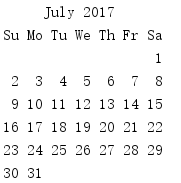import calendar
import pprint

cal = calendar.Calendar(calendar.SUNDAY)

cal_data = cal.yeardays2calendar(2020, 3)
print('len(cal_data)      :', len(cal_data))

top_months = cal_data
print('len(top_months)    :', len(top_months))

first_month = top_months
print('len(first_month)   :', len(first_month))

print('first_month:')
pprint.pprint(first_month, width=65)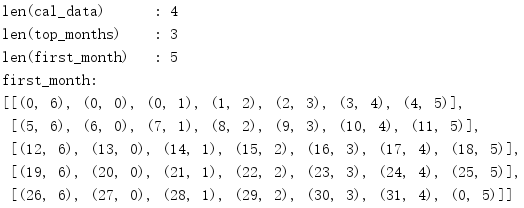import calendar

cal = calendar.TextCalendar(calendar.SUNDAY)
print(cal.formatyear(2020, 2, 1, 1, 3))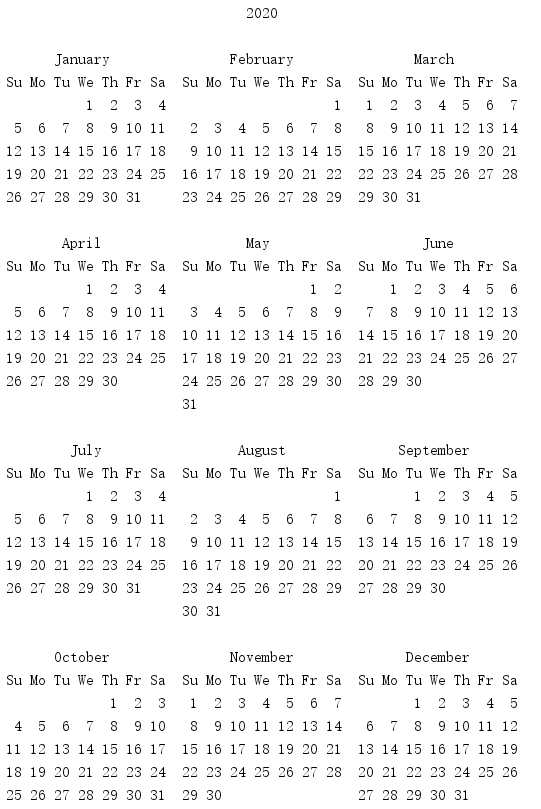## 1.2 本地化环境

import calendar

c = calendar.LocaleTextCalendar(locale='en_US')
c.prmonth(2020, 2)

print()

c = calendar.LocaleTextCalendar(locale='fr_FR')
c.prmonth(2020, 3)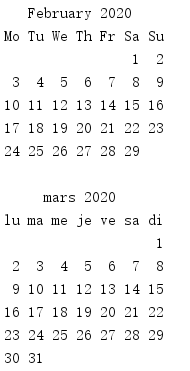## 1.3 计算日期

import calendar
import pprint

pprint.pprint(calendar.monthcalendar(2020, 7))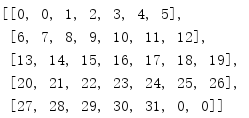posted @ 2020-03-04 19:38  SmallGrayCode  阅读(904)  评论(0编辑  收藏  举报